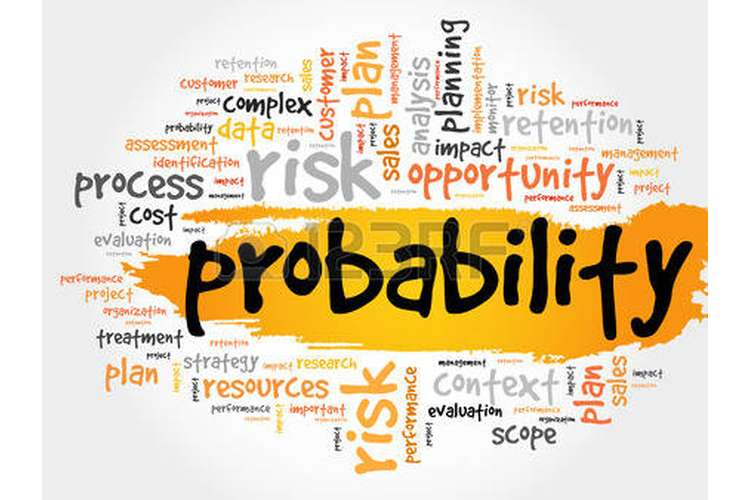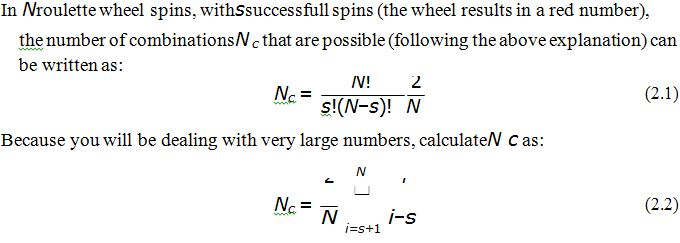ECON 360 Probability Theory and Distributions Paper Editing ServicesECON 360 Probability Theory and Distributions Paper Editing Services

In ECON 360 Probability Theory and Distributions Assignment students can clear the probability question because this assignment has different type of probability question.

Question

Below are a number of problems. You need to solve these individually, and write the solution in the report that you submit. The problems are:

A lottery game contains 49 balls, each with a number, ranging from 1 through 49. A player has to choose six numbers. When the lottery is being held, six balls from the set of balls are chosen at random.

• Calculate the probability that the player has picked all six balls correctly.
• Calculate the probability that the player has picked?ve balls correctly.
• Calculate the probability that the player has picked four balls correctly.
• Calculate the probability that the player has picked three balls correctly.
• One lottery ticket costs \$1. The payout is as follows:
• Six correct numbers: 2.5 million dollars.
• Five correct numbers: Ten thousand dollars.
• Four correct numbers: Two hundred dollars.
• Three correct numbers: Ten dollars.

Assuming a player plays the lottery 10 million times, how much can he expect to win or lose?

So, if he were to play inde?nitely, how much can he expect to win or lose for every dollar he wagers?

A blank page consists of a number of horizontal lines, located with a vertical distanceL from each other. You drop a needle of lengthLon the page. What is the probability that the needle will cross one of the lines, if the needle has fallen fully on the page? Note that the needle has to cross a line, lying on top of a line does not count as crossing it.

For Probability Help: Statistical Methods

A lunch place next to an of?ce building is open every day from 11 AM to 3 PM (4 hours). The cost to run the place is \$200 per hour. On average the shop receives 11 customers per hour, who spend on average \$20 each. On a given day, what is the probability that the shop will work with a loss?

A roulette wheel has 21 red numbers, 21 black numbers, and four zeros. A player places \$20 bets on the red numbers. If the roulette spins a red number, the player get his \$20 back, and wins another \$20. If the roulette does not spin a red number, the player loses his bet. The player sits down with \$40, and keeps on playing until he has no more money. How many spins can he expect to be able to play until he has no more money?

Some tips:

Write down the?rst couple of combinations with the lowest number of spins that will bust him. For example, the following combination of spins will bust him after two spins:

loss, loss. Because after the?rst spin he has \$20 left, after the second he is broke. The following combinations will bust him after four spins, loss, win, loss, loss, win, loss, loss, loss. In the?rst case, after the?rst spin, he has \$20. After the second spin he has \$40 again, which he loses after two more spins. In the second case, after the?rst spin, he has \$60, which he loses after three spins.Calculate the probability that the player is bust after 2 spins, after 4 spins, 6 spins, and so on, and add these probabilities up.